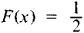# median

(redirected from median nerve injury)
Also found in: Dictionary, Thesaurus, Medical, Financial.
Related to median nerve injury: ulnar nerve injury

## median.

1 In statistics, a type of averageaverage,
number used to represent or characterize a group of numbers. The most common type of average is the arithmetic mean. See median; mode.
. In a group of numbers as many numbers of the group are larger than the median as are smaller. In the group 4, 5, 6, 9, 13, 14, 19, the median is 9, three numbers being larger and three smaller. When there is an even number of numerals in the group, the median is usually defined as the number halfway between the middle pair. 2 In geometry, the line segment connecting any vertex of a triangle to the midpoint of the opposite side; the three medians of a triangle intersect in a single point, called the median point, or centroid. The median of a trapezoid is the line segment connecting the midpoints of the nonparalled opposite sides.

## median

see MEASURES OF CENTRAL TENDENCY.
Collins Dictionary of Sociology, 3rd ed. © HarperCollins Publishers 2000
The following article is from The Great Soviet Encyclopedia (1979). It might be outdated or ideologically biased.

## Median

in geometry, the line segment joining a vertex of a triangle to the midpoint of the opposite side. The three medians of a triangle are concurrent. Their common point is called the centroid of the triangle. The centroid of a triangle cut out of material of uniform density is the center of gravity. It is also the center of gravity of a system of three equal masses situated at the vertices of the triangle. The medians of a triangle divide one another in the ratio 2:1 reckoning from the vertex to the base.

## Median

in probability theory, one of the characteristics of the distribution of values of a random variable. For a random variable X with continuous distribution function F(x), the median m is defined as the root of the equationThe random variable X assumes values larger than m with probability ½ and values smaller than m with the same probability.

In mathematical statistics, the median of an ordered sequence of n quantities x1x2 ≤ • • • ≤ xn is either xk, if n is odd and equal to 2k + 1, or (xk + xk+1)/2, if n is even and equal to 2k. As an estimate of the median for independent observations of a random variable X, we take the median of the ordered sequence constructed from the results of the observations.

## median

[′mē·dē·ən]
(mathematics)
Any line in a triangle which joins a vertex to the midpoint of the opposite side.
The line that joins the midpoints of the nonparallel sides of a trapezoid. Also known as midline.
(science and technology)
Located in the middle.
(statistics)
An average of a series of quantities or values; specifically, the quantity or value of that item which is so positioned in the series, when arranged in order of numerical quantity or value, that there are an equal number of items of greater magnitude and lesser magnitude.
McGraw-Hill Dictionary of Scientific & Technical Terms, 6E, Copyright © 2003 by The McGraw-Hill Companies, Inc.

## median

1. Biology of or relating to the plane that divides an organism or organ into symmetrical parts
2. Geometry
a. a straight line joining one vertex of a triangle to the midpoint of the opposite side
b. a straight line joining the midpoints of the nonparallel sides of a trapezium
3. Canadian the strip, often covered with grass, that separates the two sides of a highway
Collins Discovery Encyclopedia, 1st edition © HarperCollins Publishers 2005
References in periodicals archive ?
Nerve neurolysis was performed in 3 cases of median nerve injury, in 3 patients with ulnar nerve injury and in 7 with radial nerve injury.
The procedure for the induction of median nerve injury in rats, by crushing followed by stretching, led to a loss of function of the median nerve.
Long term outcome of muscle strength in ulnar and median nerve injury: comparing manual muscle strength testing, grip and pinch strength dynamometers and a new intrinsic muscle strength dynamometer.
needle that proximately caused a right median nerve injury and neuropathy" of her right arm.

Site: Follow: Share:
Open / Close